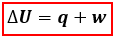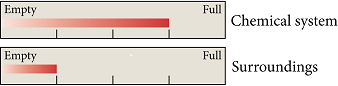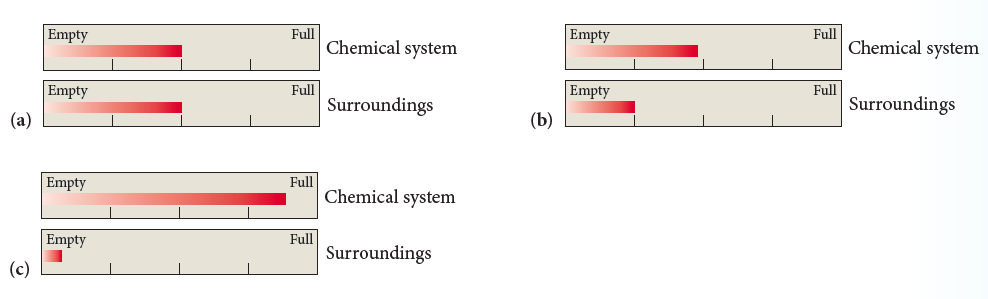# Problem: Consider these fictitious internal energy gauges for a chemical system and its surroundings:Which diagram best represents the energy gauges for the same system and surroundings following an energy exchange in which ΔEsys is negative?

###### FREE Expert Solution

The relationship between internal energy, heat, and work is shown in the following equation:ΔU = internal energy, J
q = heat, J
w = work, J

Before calculating internal energy, we have to identify heat (q) and work (w) first.

For heat, q:

+ q → if the system gains, takes in, or absorbs heat or energy
– q → if the system loses, evolves, gives off, or releases heat or energy

For work, w:

+ w → work is done on the system by the surroundings (volume: compresses)
– w → work is done by the system on the surroundings (volume: expands)

For the energy exchange given, -ΔEsys = + Δ Esurr.

96% (15 ratings)###### Problem Details

Consider these fictitious internal energy gauges for a chemical system and its surroundings:Which diagram best represents the energy gauges for the same system and surroundings following an energy exchange in which ΔEsys is negative?